# Decimation

by Ben Anderson

We now have produced highly accurate geometric models of the surface we are reconstructing.  However, we have not considered how efficient these models are.  By efficient, I mean are we representing the data using the fewest possible triangles to produce our shapes (this is important because not only does fewer triangles mean shorter rendering times, but in the case of the full human dataset, it means avoiding blowing out memory).  Unfortunately, the answer to this is a resounding no.

Consider this.  In areas of low curvature, we should only need a few triangles to represent the original shape.  In fact, the case of a flat, rectangular surface, we should only need two: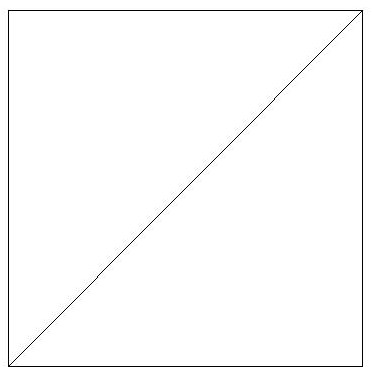However, using marching cubes, we will in fact produce two triangles for every cube intersecting this surface (because we only triangulate within the cubes):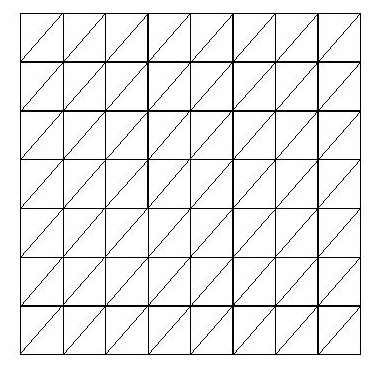This is because for every cube intersecting the surface, marching cubes must produce a triangulation.  These triangulations consist of 1-4 triangles, and thus the entire surface will maintain roughly the same triangle density regardless of curvature.  Surface grower and other triangulation methods which rely on point clouds also fall into this trap because they will triangulate the entire cloud, and unless the cloud density is lesser in areas of low curvature, triangle density will be unaffected by surface curvature.

Decimating a triangle mesh is the act of removing triangles in such a way that the overall original shape of the mesh will be maintained intact.  To do this, vertices are removed if doing so would not produce much "error" where error is defined differently depending on the type of vertex, but the basic meaning is "the amount by which removing the vertex changes the original shape."  A good decimator would take the second shape above, and turn it into the first.  Lorensen, Schroeder and Zarge identify a means of doing this:

First they classify each vertex into one of three categories:  Complex, Simple and Boundary vertices.  A complex vertex is part of multiple triangles, at least one of which contains a vertex not shared by any other triangle containing that vertex: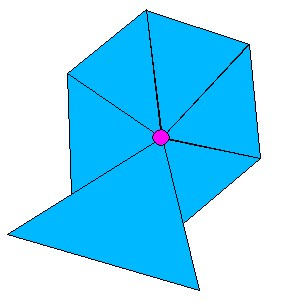A simple vertex is one completely surrounded by other triangles all of which share their outside vertices with each other: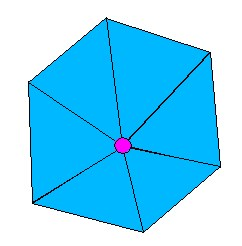A boundary vertex is one which lies on the boundary of the object (ie, on the lip of the foot in the cut-off example images):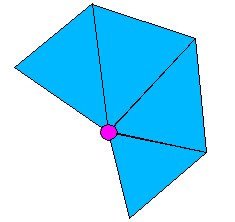Simple vertices can further be classified as interior edge vertices or corner vertices if two or more of the triangles containing them and bordering each other have particularly sharp dihedral angles compared to each other (steep is a user defined parameter).  These edges are called "feature edges" and exist when adjacent triangles come together at sharp angles.  Vertices containing two feature edges are classified as "interior edge" vertices and vertices with three or more feature edges are called "corner" vertices.

Interior edge: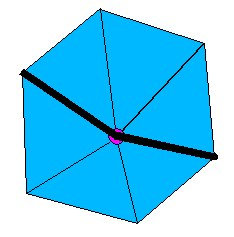Corner: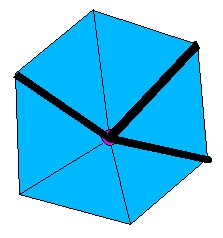Of all these classifications, only complex and corner vertices are left alone.  Complex vertices are left alone because of the difficulty in re-triangulating the whole left by removing them and the triangles they are a part of, corner vertices because they often define significant features of the shape.

Once we have classified a vertex, we must then determine the error removing it would produce.  For simple vertices, this is defined as the distance of the vertex from the "average plane" of the triangles which contain it.  The average plane is defined as the plane whose normal is the average, weighted by size, of the triangles containing the vertex, and containing the point obtained by averaging the surrounding vertices.  This looks something like: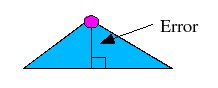The error for a boundary vertex is the distance of that point to the line defined by its neighboring boundary vertices or: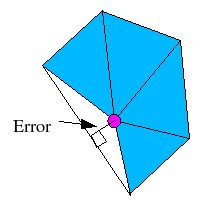And similarly, the error for an interior edge vertex is the distance of that point to the line defined by the two vertices lying on the edge: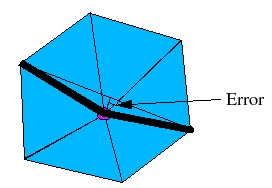Once a vertex is removed, all the triangles containing it are removed as well.  This leaves a hole which must be retriangulated.  This may be done by a variety of means, but the means used in the literature, and by our implementation is a recursive loop-splitting algorithm.  What this means is that while there are more than three vertices in the hole, two non-adjacent vertices are chosen for a split.  Then each side of the split is recursed on.  The two vertices that are chosen are those two which result in the largest "aspect-ratio" or the ratio of the distance of the closest vertex to the split plane over the length of the split line.  The split plane is the plane perpindicular to the average plane running along the split line.  Each split is also checked to make sure that all vertices on either side of the split line are on the same side of the split plane as all other vertices on that side.  For interior edge vertices, the first split is always between the two interior edge vertices.  If this all sounds complicated, it is.  Resulting retriangulations look like: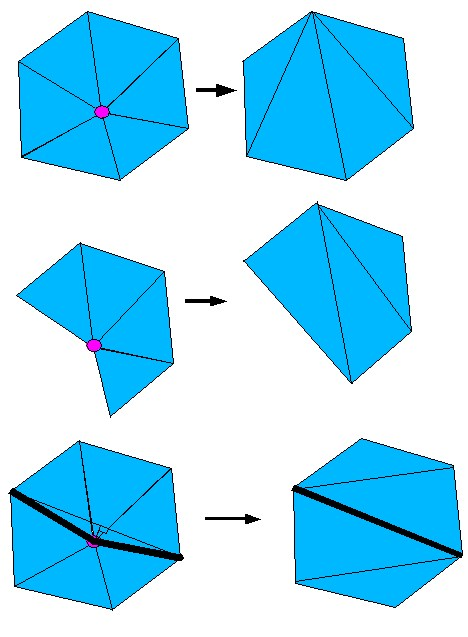We learned about decimation at almost the last minute, and as such our decimator class is somewhat incomplete.  It currently only removes simple vertices, and produces occasional artifacts in the form of missing/botched retriangulations.  It also runs relatively slowly.  However, once the artifacts are fixed, implementing both speed improvements, and removing boundary and interior edge removals are relatively easy tasks.  Here's an example of the decimator at work, and of one of its artifacts: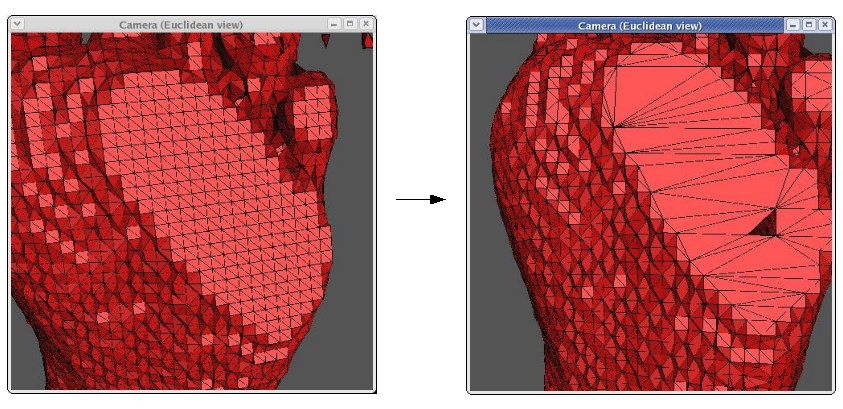You'll notice the artifacting on the bottom of the foot where the foot is missing a triangle.  The decimation stops once it moves out to the boundary and corner vertices at the edge of the flat plane, otherwise there would be fewer triangles still.  Performing a second step of decimation after smoothing also helps remove triangles (once the edges are not so sharp).  Currently, the decimator can remove roughly 50% of the triangles with minimum artifacting and no perceptible effect on overall shape.  However, due to artifacting, our final models are not currently decimated.

You might have noticed that these feet are red.